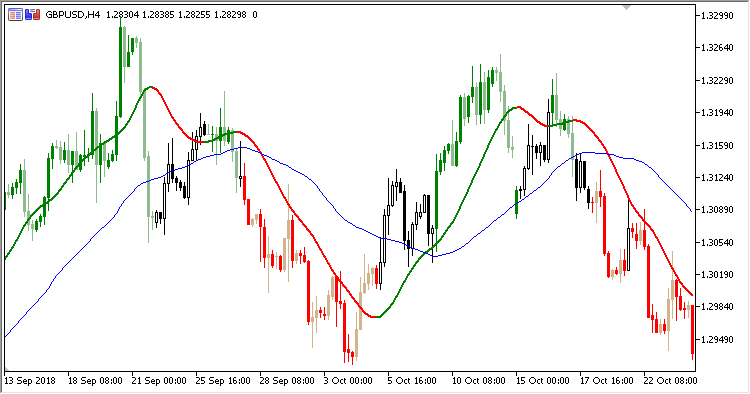• Get access

Interesting script?
So post a link to it -
let others appraise it

You liked the script? Try it in the MetaTrader 5 terminal# Slope_MA_Confirmation - indicator for MetaTrader 5

Views:
2030
Rating:
Published:
2018.11.20 12:53

Trend indicator. The indicator displays the lines and compares direction of two moving averages: Moving Average and Slope Direction Line. If the indicator lines coincide, the price chart displays colored candles.

It has six input parameters:

• MA period - Moving Average calculation period
• MA method - Moving Average calculation method
• MA applied price - Moving Average calculation price
• SDL period - Slope Direction Line calculation period
• SDL method - Slope Direction Line calculation method
• SDL applied price - Slope Direction Line calculation price
• If Moving Average and Slope Direction Line are directed upwards:
• If the candle is bullish, it is colored in green.
• If the candle is bearish, it is colored in light-green
• If Moving Average and Slope Direction Line are directed downwards:
• If the candle is bearish, it is colored in red.
• If the candle is bullish, it is colored in light-red
• In any other case, colored candles are not drawn.

Slope Direction Line indicator is not required - it is calculated by the indicator independently.Translated from Russian by MetaQuotes Software Corp.
Original code: https://www.mql5.com/ru/code/22945Slope_Direction_Line_Bar

Slope Direction Line / Moving Average confirmation bars indicatorVGridLine_Custom

The indicator plots one vertical line per day in a specified moment of timeMoving Average applied price

Now you can test the indicator and select the price type from the ENUM_APPLIED_PRICE enumerationMean_Indicator

Mean Indicator indicator